• R语言数学公式表达方式快查，方便记忆以及查询，对于数据科学研发者有好处
• 后来发现 RMarkdown （本质还是Markdown）十分的有趣，即可以写公式也可以写 R 代码，可以直接运行 R 语言，可以插入图片，直接编辑表格，并且把代码和输出结果导出到 word 、HTML 和 PDF 等。R Markdown 支持数十种...
关于为什么选择这个软件
最主要的原因是我本科就是学统计的，然后大三开始接触 Rstudio，自己也看了一些这方面的书。后来发现 RMarkdown （本质还是Markdown）十分的有趣，即可以写公式也可以写 R 代码，可以直接运行 R 语言，可以插入图片，直接编辑表格，并且把代码和输出结果导出到 word 、HTML 和 PDF 等。R Markdown 支持数十种静态和动态输出格式，包括 HTML、PDF、MS Word、Beamer、HTML5 幻灯片、Tufte 风格的讲义、书籍、仪表板、Shiny、科学文章和网站等。关于 RMarkdown 的详细介绍请查看：https://rmarkdown.rstudio.com/。这里我们只用来打公式也是可以的。
首先需要安装 Rstudio
RStudio 是 R 的集成开发环境 (IDE)，它包括一个控制台、支持直接执行代码的语法高亮编辑器，以及用于绘图、历史、调试和工作空间管理的工具。RStudio 有开源版和商业版，可在桌面上（Windows、Mac 和 Linux）或连接到 RStudio Server 或 RStudio Server Pro（Debian/Ubuntu、Red Hat/CentOS 和 SUSE Linux）的浏览器中运行。
安装 knitr 和 rmarkdown 包
新版本的 Rstudio 已经默认安装了这两个包，检验自己的 Rstudio 是否已经安装了这两个包的方法十分简单，可以直接点击 File–New File–Rmarkdown,如果可以成功创建就说明已经有 rmarkdown 这个包了。点击上方的 Knit 等待几秒钟，如果成功导出 word 那么就不需要安装 knitr 包了。具体创建和导出步骤见下一段：
创建 RMarkdown 和导出文档
点击左上角的 File–New File–Rmarkdown
在弹出的对话框选择 word（十分建议选择 word，中文 PDF 十分繁琐，如果只使用纯英文可以选择 PDF，HTML 如果有网站需求可以选择），Title 和 Author 都可以不写，建议写个标题。选择完成之后点击 OK 就可以创建一个 RMarkdown 文件了。点击左上角的保存，选择自己想要保存的位置，给文件命名，如果中间弹出选择保存编码格式，请选择 UTF-8 否则中文会乱码。
Rmarkdown 包括的内容
下面的图是创建的 RMarkdown 默认包括的内容，本着实用的角度我们不过多介绍这些内容的详细信息。红色箭头所指的是我们现在正在编辑的文档，黄色的箭头是 R 代码块，绿色的箭头指向的是标题（一个#是一级标题，两个#就是二级标题以此类推），蓝色箭头所指的是正文。最上面的六行是文件中的 2、3 和 4 行都是一些文档的信息可以删除，第5行最好不要删除。第 8 行到第 10 行是检查 knitr 包初始化检测，如果在文章中不需要使用 R 语言的代码块，这个也可以删掉。下面的内容就是个例子，直接删除就可以。RMarkdown 编写公式
在创建完成 RMarkdown之后，就可以编写了。插入公式和 LaTeX 几乎没有区别，两个美元符号 $$ 中间就是编写行内公式（公式和句子在同一行），四个美元符号 $$$$ 中间就是行间公式（公式单独一行）。 简单的例子：\hat y = \hat \beta_0 + \hat \beta_1 X_1 + \hat \beta_2 X_2 上面的行内公式输出效果： y ^ = β ^ 0 + β ^ 1 X 1 + β ^ 2 X 2 \hat y = \hat \beta_0 + \hat \beta_1 X_1 + \hat \beta_2 X_2 这就是回归方程的公式。 一个复杂点的例子： $$ \begin{equation}\begin{split} c_B(t,s)=& E[B_tB_s]-E[B_t]E[B_s]\\ =& E[[(B_t-B_s)+B_s]B_s]\\ =& E[(B_t-B_s)B_s]+EB_s^2\\ =& E(B_t-B_s)EB_s+s\\ =& 0+s=s,\ 0\leq s<t \end{split}\end{equation} $$ 这个行间公式的输出效果见下面的截图。这是求布朗运动协方差的一种方法的步骤。$$\mathbb {F} \mathcal {F}
上面的是两种常用的花体写法，效果：

F

F

\mathbb {F} \mathcal {F}

还有很多公式方面的内容，可以直接查看 LaTeX 官方文档 https://www.latex-project.org/help/documentation/，或者百度关键字查找，LaTeX 中希腊字母、上标和下标、括号、求和与积分、分式与根式、多行表达式、特殊函数与符号等等。 目前我也没有很深入的研究，知道这些就可以开始编写公式了。
导出文档
导出文档可以使用代码导出：
knitr::knit("dome.Rmd","C:/Users/Desktop/dome.dock")

这样就可以将 word 文档导出到桌面上了。 也可以直接点击 Knit 导出，我们这里想要尽快的使用 Rmarkdown 因此选择直接点解 Knit（这样比较简单，不需要学习 R 代码）。如果前面选择了 word 就可以直接点击红色箭头所指的 Knit 导出 word，如果想要导出其他的类型可以点击黄色箭头所指的小三角，这样就可以选择的想要导出的文档类型，包括 word 、PDF 和 HTML 等等。可以看到打完公式之后下面就直接出现公式的效果，如果打完公式下面没有出现这种效果，说明公式存在问题，需要仔细检查问题。可以看到导出的 word 是这样的，这些公式都是可以直接复制到 PPT 等地方的，有了 word 就很容易得到 PDF了，而且中文的 PDF 导出并不简单（全英文的直接点击 knit 就可以，中文的需要安装一个新的包），这里建议导出 word。有了这些就可以使用 RMarkdown 编辑公式了，下面的内容可以不看了，但是如果想要插入图片等等操作，请继续往下看。
关于 RMarkdown 独特的一些东西
RMarkdown 之所以可以使用 LaTeX 的方式写公式，本质上是因为使用了支持 LaTeX 语法的包。下面这个截图通过选择工具栏 Tools–Global Options–Sweave 可以看到蓝色小箭头所指的部分有两个选项，都是 …LaTeX （这里建议选择 xeLaTeX）这说明很可能 LaTeX 已经变成这个软件的一个包了，其中 xeLaTeX 就是 谢益辉把 LaTeX做了一点修改，将其变成了 R 的一个包 (package).插入图片
下面的图中是插入图片方法，第一个包括三部分，首先有一个英文的 !；然后是一个英文的[],方括号中可以写文字，这个文字可以是图片的名称导出 word 之后这个名称会在图片的下方显示；第三部分是图片的路径加名称，这里我只写了图片的名称是因为我的图片和这个 rmarkdown 文件在一个文件夹下。在这里 ![](C:/Users/sparrow/Desktop/RMarkdown教程/图片演示.png) 这样写可以和![](图片演示.png) 的效果相同。
插入网址
插入网址的方式和插入图片相似，插入网址有两种方式。[CTeX](http://ctex.org)这样就可以插入一个网址了，方括号可是网址的名字，但是如果方括号里面有字，那么导出之后就不会打印网址，但是网址会以超链接的形式存在。使用 <https://rmarkdown.rstudio.com/lesson-3.html> 也可以插入网址，这样网址会直接打印出来。
直接显示公式效果
插入 R 代码块
这部分对于不使用 R 语言的不需要了解, 点击右上角的 Insert 点击 R 就可以插入一个 R 代码块了,可以在代码块中间插入代码，对 R 语言感兴趣的可以探索一下。PDF 若干问题
导出 PDF 时保存 tex 文档
在导出 PDF 时可以保存 tex 文档,这里红色箭头所指的地方，可以填多个，这样就是即导出 word 又导出 PDF，在 pdf_document: 下面添加 keep_tex: yes，就可以在导出 PDF 时保留 tex 文档。中文PDF
关于中文 PDF 有推荐看谢益辉的解决方案：https://yihui.org/tinytex/cn/.
论文模板
下载 rticles 包，就可以使用其他的模板了。
使用步骤 File–New File–Rmarkdown–From Template 可以选择模板包括 IEEE 等多种模板。目前的不足
在论文排版方面是不如 LaTeX 的，字体以及大小缩进可以百度查到，我还没有验证这里就不写了。建议导出 word 之后在 word 中调格式，但是修改完 word 之后记得另存重命名，不然下次 knit 之后又会覆盖掉。该简单版教程仅代表个人使用过程中的一点经验和看法，可能存在一些错误，希望各位可以指出。
另外如果转载请注明出处。 以百度查到，我还没有验证这里就不写了。建议导出 word 之后在 word 中调格式，但是修改完 word 之后记得另存重命名，不然下次 knit 之后又会覆盖掉。该简单版教程仅代表个人使用过程中的一点经验和看法，可能存在一些错误，希望各位可以指出。
另外如果转载请注明出处。
展开全文• 想用R语言复现图中公式，输入应该是某些因子的多列1到N的排序顺序，输出应该是基于这些排序通过这个公式计算后的最终排序结果 目前没有思路，希望大家提提建议算法 有问必答
• ## R语言作图：数学公式

万次阅读 多人点赞 2018-02-07 18:44:07
在使用R语言作图时，有时需要在图上标注诸如求和、积分、上下标等数学符号，该操作可以通过expression函数完成。 　expression(...) 　括号中输入数学表达式，配合plot、text、title、axis等函数使用，可以将数学...
　　在使用R语言作图时，有时需要在图上标注诸如求和、积分、上下标等数学符号，该操作可以通过expression函数完成。
expression(...)
括号中输入数学表达式，配合plot、text、title、axis等函数使用，可以将数学公式绘制在图表上。

x <- seq(0, 4, 0.01)
y <- sqrt(x)
tt <- expression(y == sqrt(x))
plot(x, y, type = 'l', main = tt, las = 1)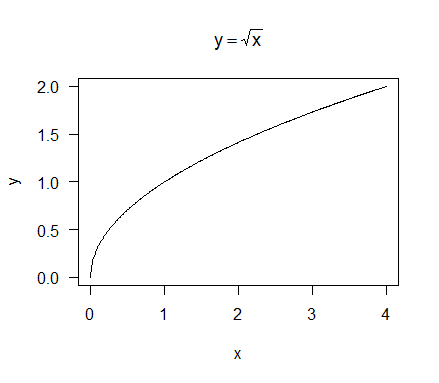expression函数支持的表达式语法与Latex类似，常用的表达式语句可以查看plotmath的帮助，即?plotmath。下面简单总结一下：

1、基础运算符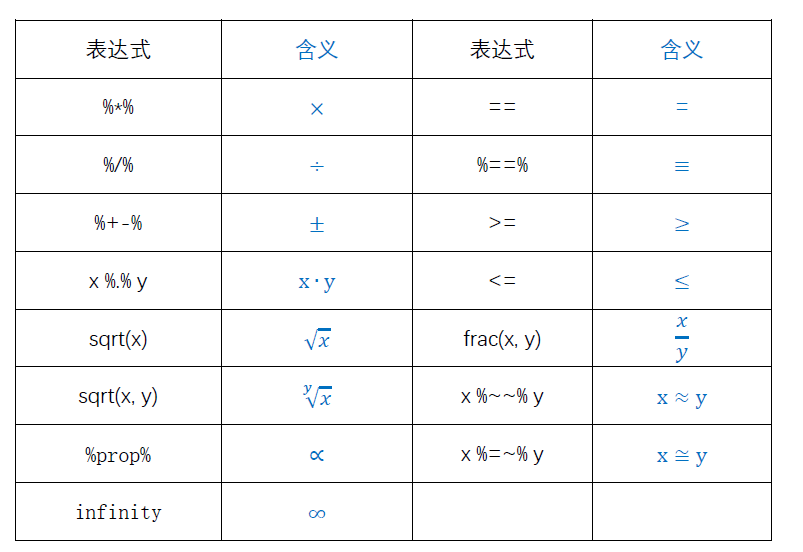2、大型运算符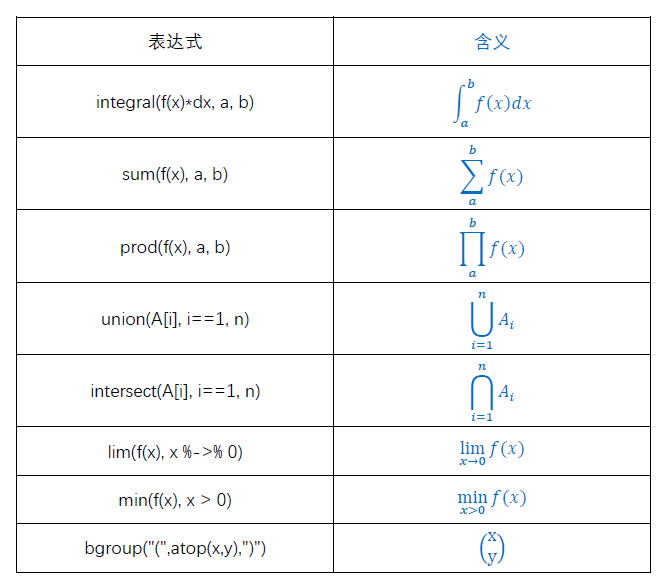3、上下标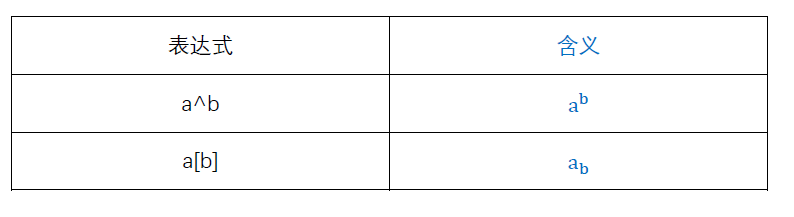4、箭头符号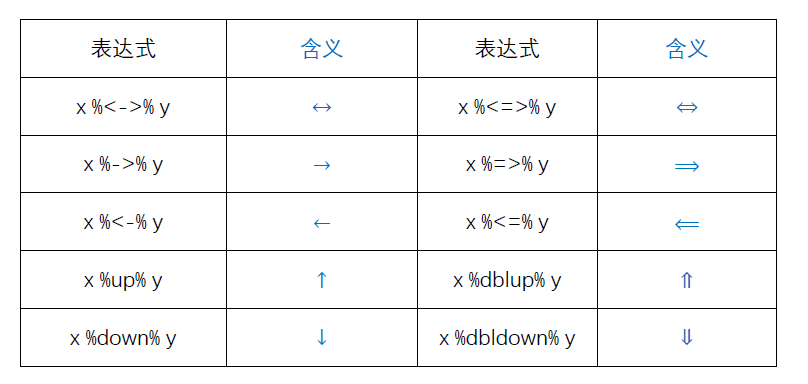5、集合运算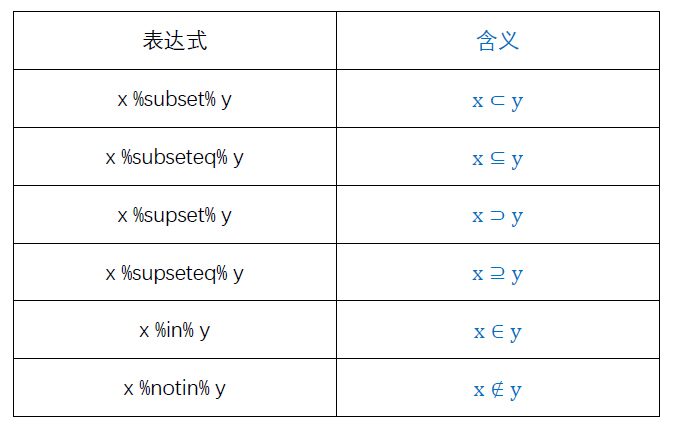6、导数符号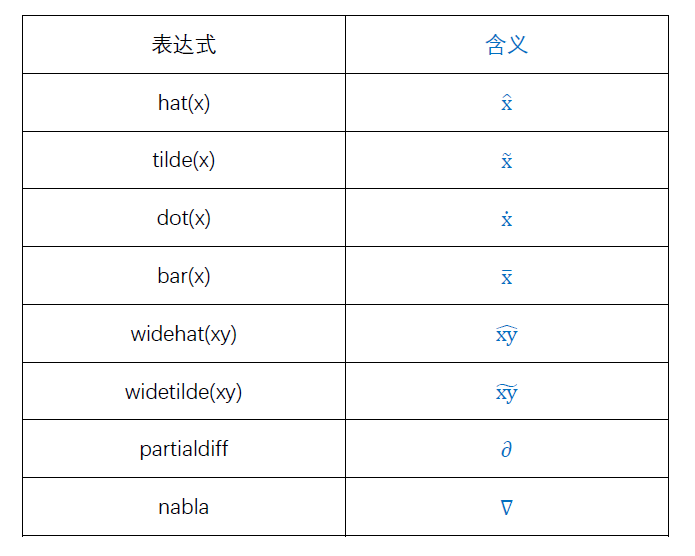7、特殊字体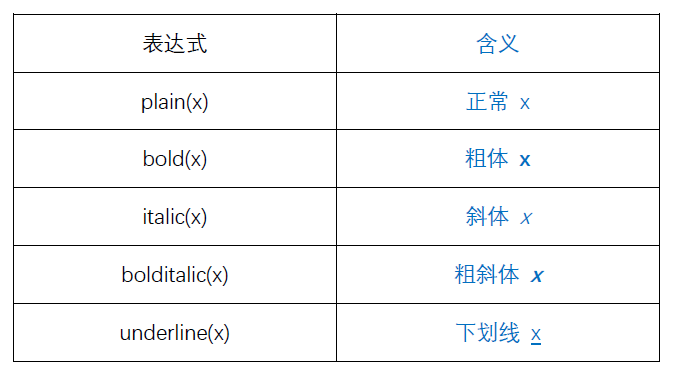8、希腊字母

希腊字母既可以使用其英文名表达，也可以使用symbol()表达。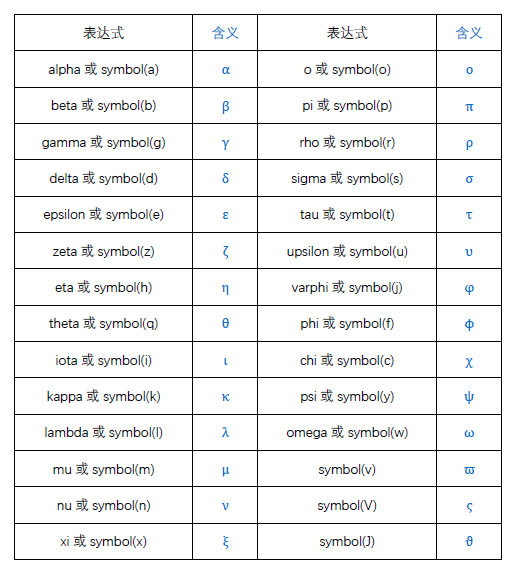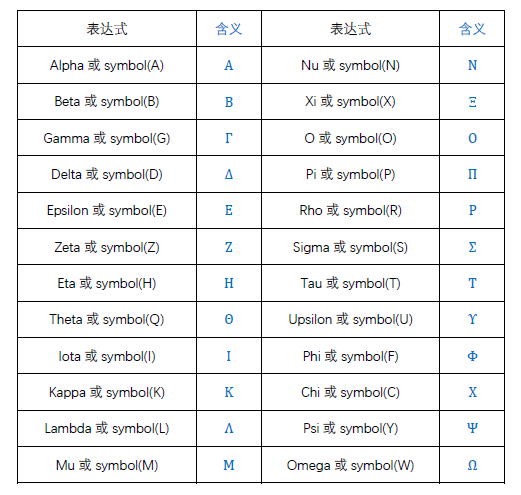展开全文数学符号 数学表达式 可视化
• 协方差的计算公式R语言进行验证 首先附上协方差公式： 来设5个样本点：（3,9），（2,7），（4,12），（5,15），（6,17） 用R绘制出散点图，大概是这样： 要求这5个点的协方差，首先样本点为5个，n=5，X依次取3,2...
协方差的计算公式及R语言进行验证
首先附上协方差公式：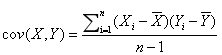来设5个样本点：（3,9），（2,7），（4,12），（5,15），（6,17）
用R绘制出散点图，大概是这样：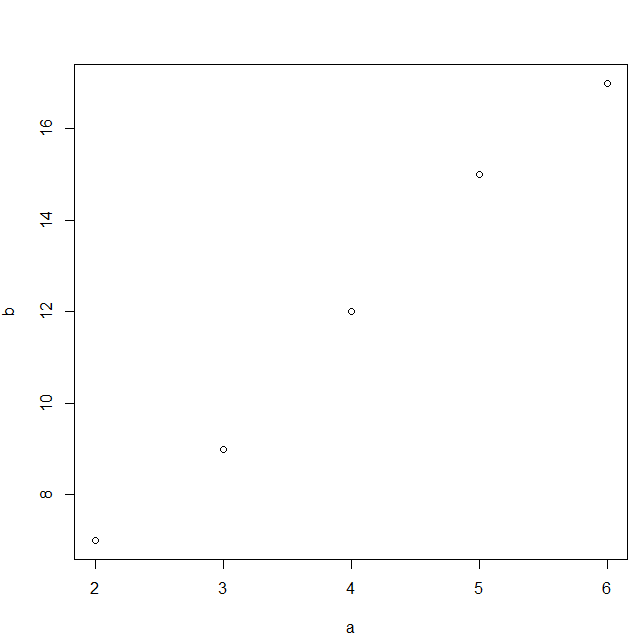要求这5个点的协方差，首先样本点为5个，n=5，X依次取3,2,4,5,6，Y依次取9,7,12,15,17。X的均值为4，带入公式可得：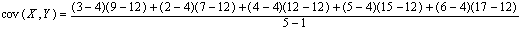不难计算出结果为6.5
现在用R语言进行验证：
已知R语言里边协方差函数为cov(x,y) 我们分别用cov()函数和上述公式来进行仿真结果，代码如下：
a <- c(3,2,4,5,6)
b <- c(9,7,12,15,17)
COV=0
EX=mean(a)
EY=mean(b)
for(j in 1:5){
COV <- COV+(a[j]-EX)*(b[j]-EY)/4
}
COV
cov(a,b)

输出结果如下：
> COV
 6.5
> cov(a,b)
 6.5

由此可得，计算公式得出的结果完全正确
展开全文协方差
• R语言的绘图函数中，如果文本参数是合法的R语言表达式，那么这个表达式就被用Tex类似的规则进行文本格式化。y (x) (exp(-(x^2)/2))/sqrt(2*pi)plot(y, -5, 5, main = expression(f(x) == frac(1,sqrt(2*pi))*e^(-...
  在R语言的绘图函数中，如果文本参数是合法的R语言表达式，那么这个表达式就被用Tex类似的规则进行文本格式化。
y <- function(x) (exp(-(x^2)/2))/sqrt(2*pi)

plot(y, -5, 5, main = expression(f(x) == frac(1,sqrt(2*pi))*e^(-frac(x^2,2))), lwd = 3, col = "blue")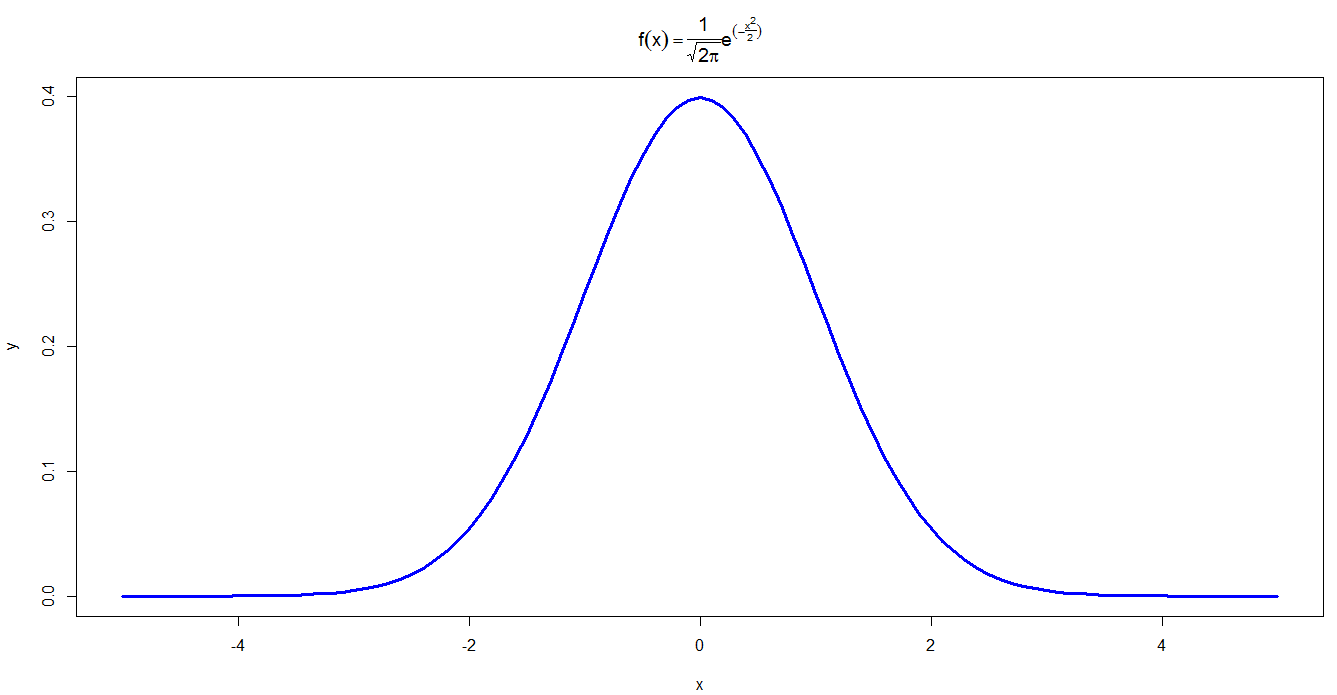library(ggplot2)

x <- seq(0, 2*pi, by = 0.01)
y <- sin(x)
data <- data.frame(x, y)
p <- ggplot(data, aes(x, y)) +　geom_line()

p + geom_area(fill = 'blue', alpha = 0.3) +
scale_x_continuous(breaks = c(0, pi, 2*pi), labels = c('0', expression(pi), expression(2*pi))) +
geom_text(parse = T, aes(x = pi/2,y = 0.3, label = 'integral(sin(x)*dx, 0, pi)'))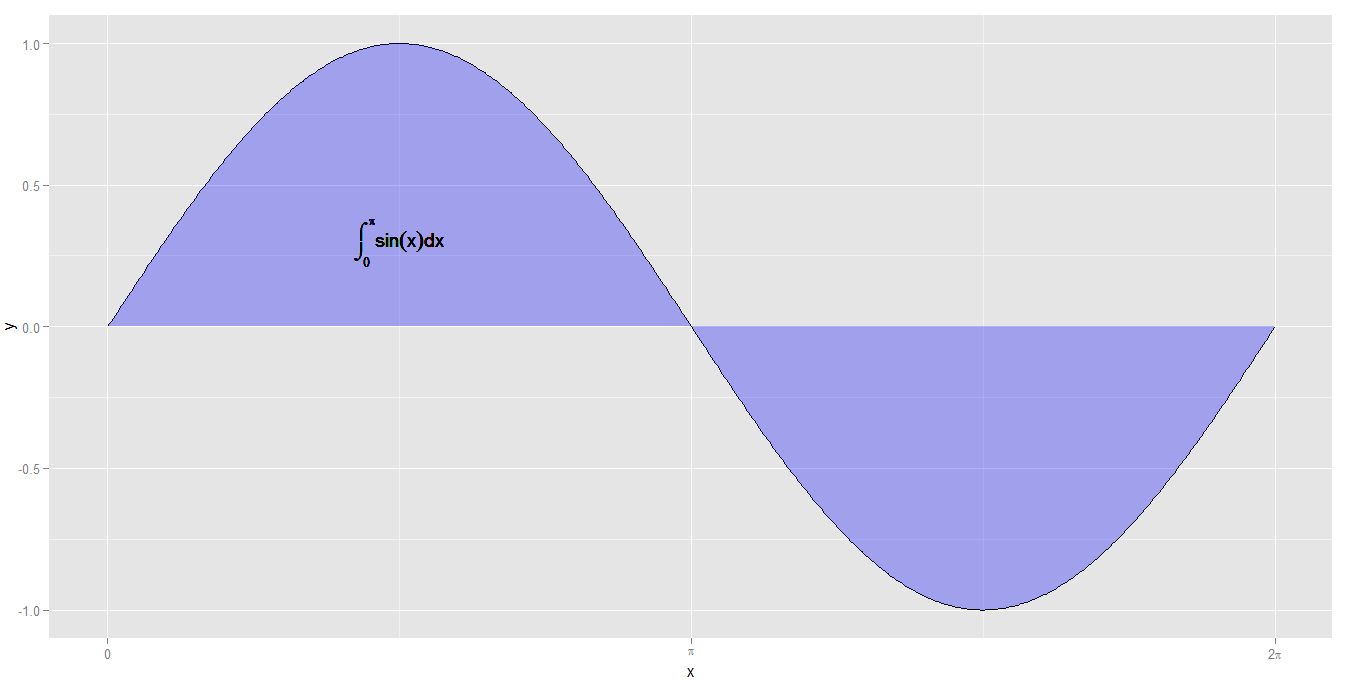##一、R语言的“表达式”
在R语言中，“表达式”的概念有狭义和广义两种意义。狭义的表达式指表达式（expression）类对象，由expression函数产生；而广义的的表达式既包含expression类，也包含R“语言”类（language）。expression和language是R语言中两种特殊数据类：

getClass(“expression”)

# Class "expression" [package "methods"]

# No Slots, prototype of class "expression"

# Extends: "vector"


getClass(“language”)

# Virtual Class "language" [package "methods"]

# No Slots, prototype of class "name"

# Known Subclasses:
# Class "name", directly
# Class "call", directly
# Class "{", directly
# Class "if", directly
# Class "<-", directly
# Class "for", directly
# Class "while", directly
# Class "repeat", directly
# Class "(", directly
# Class ".name", by class "name", distance 2, with explicit coerce

可以看到expression类由向量派生得到，而language类是虚拟类，它包括我们熟悉的程序控制关键词/符号和name、call 子类。
##二、产生“表达式”的函数
虽然我们在R终端键入的任何有效语句都是表达式，但这些表达式在输入后即被求值（evaluate）了，获得未经求值的纯粹“表达式”就要使用函数。下面我们从函数参数和返回值两方面了解expression、quote、bquote和substitute这几个常用函数。
####1、expression 函数
expression函数可以有一个或多个参数，它把全部参数当成一个列表，每个参数都被转成一个表达式向量，所以它的返回值是表达式列表，每个元素都是表达式类型对象，返回值的长度等于参数的个数：
(ex <- expression(x = 1, 1 + sqrt(a)))

## expression(x = 1, 1 + sqrt(a))

length(ex)

##  2

ex

## expression(x = 1)

mode(ex)

##  "expression"

typeof(ex)

##  "expression"

ex

## expression(1 + sqrt(a))

mode(ex)

##  "expression"

typeof(ex)

##  "expression"

因为expression函数把参数当成列表处理，所以等号‘=’两边的表达式要符合R语言列表元素的书写规则，否则出错，比如：
expression(x+11=1)

####2、quote函数
quote函数只能有一个参数。quote函数的返回值一般情况下是call类型，表达式参数是单个变量的话返回值就是name类型，如果是常量那么返回值的存储模式就和相应常量的模式相同：
(cl <- quote(1 + sqrt(a) + b^c))
## 1 + sqrt(a) + b^c

mode(cl)
##  "call"

typeof(cl)
##  "language"

(cl <- quote(a))
## a

mode(cl)
##  "name"

typeof(cl)
##  "symbol"

(cl <- quote(1))
##  1

mode(cl)
##  "numeric"

typeof(cl)
##  "double"

quote返回值如果是name或常量类型，它的长度就是1；如果是call类型，返回值长度就与函数/运算符的参数个数n对应，长度等于n+1，多出的长度1是函数/符号名。
length(quote(a))  #name或常量类型，返回值长度为1
##  1

length(quote(!a))  #单目运算符，返回值长度为2
##  2

length(quote(-b))  #单目运算符，返回值长度为2
##  2

length(quote(a + b))  #双目运算符，返回值长度为3
##  3

length(quote((a + b) * c))  #多个运算符只算优先级最低的一个
##  3

####3、bquote 和 substitute 函数
如果不使用环境变量或环境变量参数，bquote 和 substitute 函数得到的结果与quote函数相同。
bquote(1 + sqrt(a) + b^c) == quote(1 + sqrt(a) + b^c)
##  TRUE

substitute(1 + sqrt(a) + b^c) == quote(1 + sqrt(a) + b^c)
##  TRUE

但是bquote 和 substitute 函数可以在表达式中使用变量，变量的值随运行进程而被替换。bquote 和 substitute 函数变量替换的方式不一样，bquote函数中需要替换的变量用 .( ) 引用，substitute函数中需要替换的变量用列表参数方式给出。除了这一点，bquote 和 substitute 函数没有差别：
a <- 3

b <- 2

(bq <- bquote(y == sqrt(.(a), .(b))))
## y == sqrt(3, 2)

(ss <- substitute(y == sqrt(a, b), list(a = 3, b = 2)))
## y == sqrt(3, 2)

bq == ss
##  TRUE

搞出两个功能完全一样的函数不算很奇怪，R语言里面太多了，可能是照顾不同使用习惯的人们吧。bquote函数的帮助档说这个函数类似于LISP的backquote宏，对于像我这样的LISP盲，使用substitute函数好一些。 substitute函数的典型用途是替换表达式中的变量，如果我们希望在表达式中使用变量并且希望这些变量在运行过程中做出相应改变，就可以使用substitute函数。
par(mar = rep(0.1, 4), cex = 2)

plot.new()

plot.window(c(0, 10), c(0, 1))

for (i in 1:9) text(i, 0.5, substitute(sqrt(x, a), list(a = i + 1)))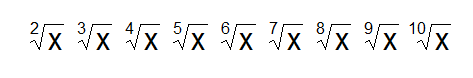####4、parse 函数
parse函数用于从文件读取文本作为表达式，返回的值是expression类型，这函数也很有用。后面有例子。
x <- 1

x + "x"

## Error: 二进列运算符中有非数值参数

expression(x + "x")

## expression(x + "x")

quote(x + "x")

## x + "x"

但R要检查表达式中的运算符，不符合运算符使用规则的表达式将出错：
expression(x + +++y)
## expression(x + +++y)

expression(x - ---y)
## expression(x - ---y)
## expression(x****y) (Not run) expression(xy) (Not run)
## expression(1<=x<=4) (Not run)

quote(x + +++y)
## x + +++y

quote(x - ---y)
## x - ---y
## quote(x****y) (Not run) quote(xy) (Not run) quote(1<=x<=4) (Not run)

+ - 运算连续使用不出错是因为它们还可以当成求正/负值运算的符号。 在表达式产生函数中使用paste函数可以解决这样的问题。在这种条件下，paste对参数的处理方式和表达式产生函数一样，检查运算符但不检查变量名。用NULL作为运算符的参数可以获得意外的效果：
ex <- expression(paste(x, "", y))

cl <- quote(paste(x, "****", y))

par(mar = rep(0.1, 4), cex = 2)

plot.new()

plot.window(c(0, 1.2), c(0, 1))

text(0.2, 0.5, ex)

text(0.6, 0.5, cl)

cl <- quote(paste(1 <= x, NULL <= 4))

text(1, 0.5, cl)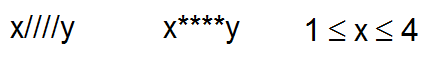##三、R绘图函数对文本参数中的表达式的处理
quote, bquote 和 substitute 的返回值有三种类型call, name 和 常量，事实上expression 函数的结果最终也是这三种类型。因为expression函数的结果是expression列表，我们取列表元素的值检查看看：
(ex <- expression(1 + sqrt(x), x, 1))
## expression(1 + sqrt(x), x, 1)

ex[]
## 1 + sqrt(x)

mode(ex[])
##  "call"

typeof(ex[])
##  "language"

ex[]
## x

mode(ex[])
##  "name"

typeof(ex[])
##  "symbol"

ex[]
##  1

mode(ex[])
##  "numeric"

typeof(ex[])
##  "double"

确实是这样。所以绘图函数对文本参数中的表达式处理就有三种情况。先看看处理结果：
par(mar = rep(0.1, 4), cex = 2)

plot.new()

plot.window(c(0, 1.2), c(0, 1))

text(0.2, 0.5, ex)

text(0.6, 0.5, ex)

text(1, 0.5, ex)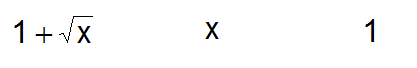name 和常量类型都很简单，直接输出文本，而call类型就不好判断了。我们前面说过call类型返回值的长度与函数/运算符的参数个数有关。这是怎么体现的呢？由于文本参数最终得到的是文本，我们用as.character函数来看看：
as.character(quote(x - y))
##  "-" "x" "y"

as.character(quote(1 - x + y))
##  "+"  "1 - x" "y"

as.character(quote((1 + x) * y))
##  "*"  "(1 + x)" "y"

as.character(quote(!a))
##  "!" "a"

as.character(quote(sqrt(x)))
##  "sqrt" "x"

转换成字符串向量后排在第一位的是运算符或函数名称，后面是参数（如果参数中还有运算符或函数名，R还会对其进行解析）。运算符和函数是相同的处理方式。事实上，在R语言中，所有运算符（包括数学运算符和逻辑运算符）都是函数，你可以用函数的方式使用运算符：
2 + 4
##  6

2 - 4
##  -2

2 <= 4
##  TRUE

2 >= 4
##  FALSE

R绘图函数对表达式中包含的函数名和它们的参数首先应用Tex文本格式化规则进行处理，这种规则的具体情况可以使用 ?plotmath 进行查看，主要是一些数学公式和符号的表示方法。把这个说明文档中字符串拷贝到maths.txt文件中并保存到当前工作目录后可以用下面的代码做出后面的表格：
ex <- parse("maths.txt")

n <- length(ex)

par(mar = rep(0.1, 4), cex = 0.8)

plot.new()

plot.window(c(0, 8), c(0, n/4))

y <- seq(n/4, by = -1, length = n/4)

x <- seq(0.1, by = 2, length = 4)

xy <- expand.grid(x, y)

text(xy, labs, adj = c(0, 0.5))

xy <- expand.grid(x + 1.3, y)

text(xy, ex, adj = c(0, 0.5), col = "blue")

box(lwd = 2)

abline(v = seq(1.3, by = 2, length = 4), lty = 3)

abline(v = seq(2, by = 2, length = 3), lwd = 1.5)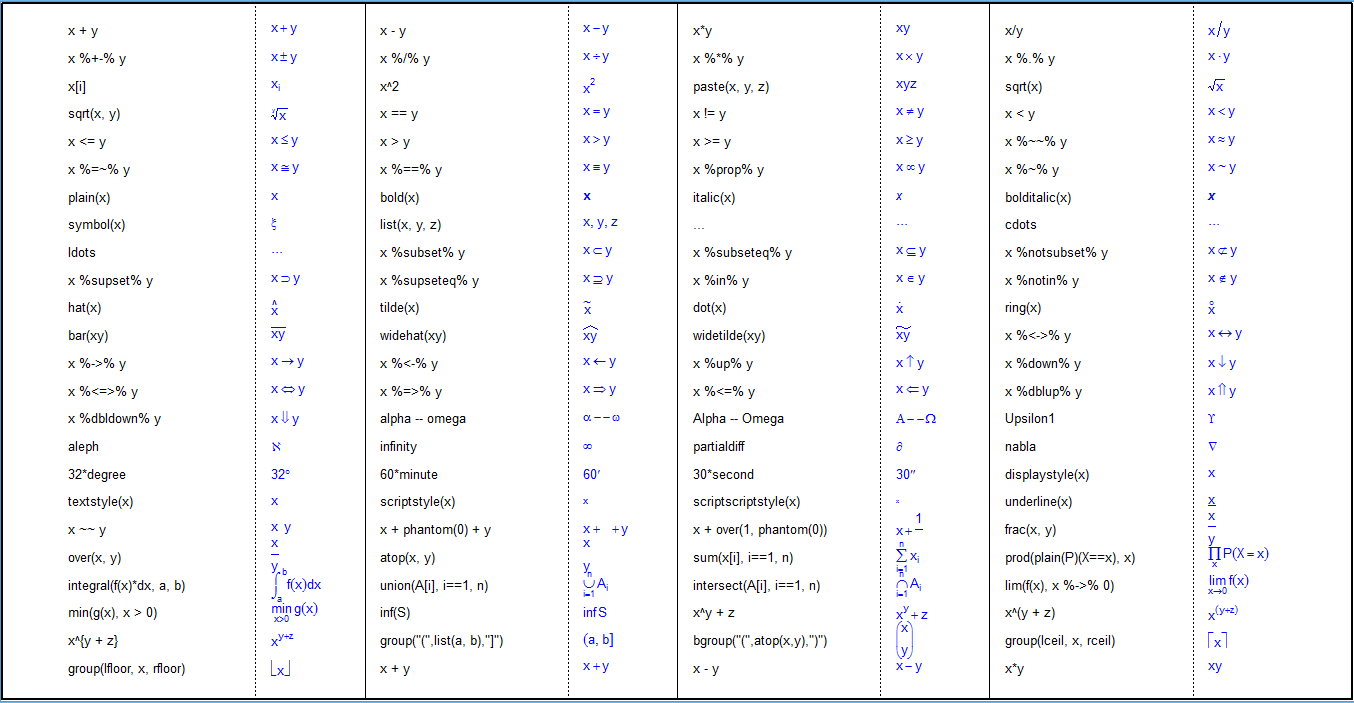右键查看图片，浏览大图
表中奇数列是字符串（表达式），偶数列（蓝色）是Tex格式化的图形。除了上表列出的规则外还有一些拉丁文和希腊文符号，可以在表达式中用 symbol 函数或名称（如alpha）等表示，用到时自己去找吧。 如果函数名（包括运算符）有对应的Tex格式化规则，函数名和参数都按规则进行图形绘制；如果没有，就当成是R语言普通函数：
ex <- expression(sqrt(x), x + y, x^2, x %in% A, x <= y, mean(x, y, z), x | y, x & y)

n <- length(ex)

par(mar = rep(0.1, 4), cex = 1.5)

col <- c("red", "blue")

plot.new()

plot.window(c(0, n), c(0, 1))

for (i in 1:n) text(i - 0.5, 0.5, ex[i], col = col[i%%2 + 1])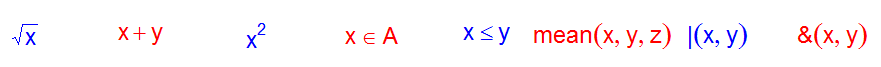上面例子中前5种运算函数都是有对应数学符号的，所以它出的图（符号和顺序）与数学习惯一致，后三种运算函数没有对应数学符号，所以用普通函数方式（函数名在前，参数在括号内用逗号分隔）出图。其他还有一些琐碎的规则，自己找找吧。
本文转自科学网李鹏程博客

欢迎访问我的个人站点：http://bgods.cn/

展开全文• #####模型和公式##### #利用base包的cars数据集来讲解模型和公式这一章 cars.lm(formula=dist~speed,data=cars) cars.lm #其中的lm是估算线性模型参数的函数,formula代表公式,dist~speed就是公式,cars是数据集 #使用...
• 方法一直接利用expression 方法二利用latex2exp latex2exp包实际上是一种将latex语法转变为expression语句的辅助包。 install.packages('latex2exp') library(latex2exp) ...plot(1,1,main= TeX('$\\alpha^\\beta$'...作图
• 实际开发中发现，JS先加载，VUE再获取数据，这样，公式就显示不出来了。 解决方法：设置页面2S后再加载JS 2、页面翻页后，第二页的公式渲染不出来 原因同上，JS已经在再过一遍了。 解决方法，在链接上加一个page...
• 在做统计回归实验作业的时候老师要求将R语言程序输出转化为数学公式写到报告里 比如 将这个结果转化成 这个样子，如果变量很多的话就需要码很多字非常麻烦，于是我写了一个python程序，只要把 (Intercept) -3.690e...python latex 逻辑回归
• r语言中将字符串转化为公式When you open an Excel worksheet or change any entries or formulas in the worksheet, Excel automatically recalculates all the formulas in that worksheet by default. This can ...字符串 excel java 大数据 机器学习...